# RD Sharma Class 10 Solutions Chapter 3 Pair of Linear Equations in Two Variables Ex 3.8

## RD Sharma Class 10 Solutions Chapter 3 Pair of Linear Equations in Two Variables Ex 3.8

These Solutions are part of RD Sharma Class 10 Solutions. Here we have given RD Sharma Class 10 Solutions Chapter 3 Pair of Linear Equations in Two Variables Ex 3.8

Other Exercises

Question 1.
The numerator of a fraction is 4 less than the denominator. If the numerator is decreased by 2 and denominator is increased by 1, then the denominator is eight times the numerator. Find the fraction. (C.B.S.E. 1990)
Solution:
Let numerator of a fraction = x
and denominator = y
Then fraction = $$\frac { x}{ y}$$
According to the conditions,
y – x = 4 ….(i)
and 8 (x – 2) = y + 1
=> 8x – 16 – y + 1
=> 8x – y = 1 + 16
=> 8x – y= 17 ….(ii)
7x = 21 => x = 3
y – 3 = 4
=> y = 4 + 3 = 7
Hence fraction = $$\frac { x}{ y}$$

Question 2.
A fraction becomes $$\frac { 9 }{ 11 }$$ if 2 is added to both numerator and the denominator. If 3 is added to both the numerator and the denominator, it becomes $$\frac { 5 }{ 6 }$$. Find the fraction. (C.B.S.E. 1990)
Solution:
Let the numerator of a fraction = x
and denominator = y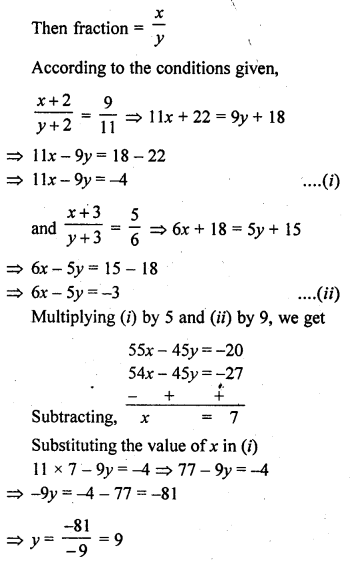Fraction = $$\frac { x}{ y}$$ = $$\frac { 7 }{ 9 }$$

Question 3.
A fraction becomes $$\frac { 1 }{ 3 }$$ if 1 is subtracted from both its numerator and denominator. If 1 is added to both the numerator and denominator, it becomes $$\frac { 1 }{ 2 }$$. Find the fraction. (C.B.S.E. 1993C)
Solution:
Let the numerator of a fraction = x
and denominator = y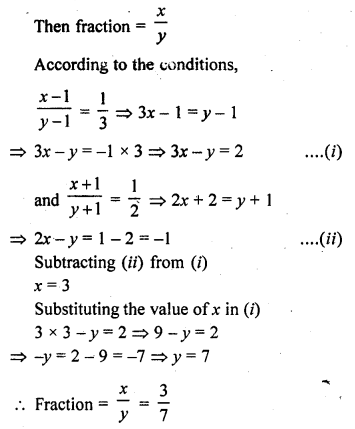Question 4.
If we add 1 to the numerator and subtract 1 from the denominator, a fraction becomes 1. It also becomes $$\frac { 1 }{ 2 }$$ if we only add 1 to the denominator. What is the fraction.
Solution:
Let numerator of a fraction = x
and denominator = y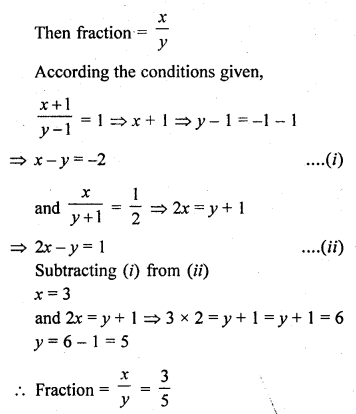Question 5.
The sum of the numerator and denominator of a fraction is 12. If the denominator is increased by 3, the fraction becomes $$\frac { 1 }{ 2 }$$. Find the fraction. (C.B.S.E. 2006C)
Solution:
Let the numerator of a fraction = x
and denominator = y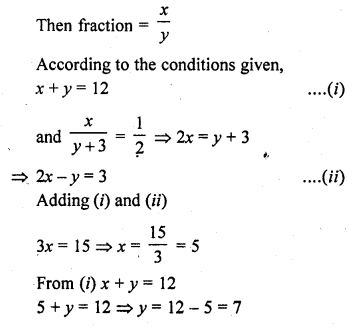Fraction = $$\frac { x}{ y}$$ = $$\frac { 5 }{ 7 }$$

Question 6.
When 3 is added to the denominator and 2 is subtracted from the numerator a fraction becomes $$\frac { 1 }{ 4 }$$. And, when 6 is added to numerator and the denominator is multiplied by 3, it becomes $$\frac { 2 }{ 3 }$$. Find the fraction.
Solution:
Let numerator of a fraction = x
and denominator = y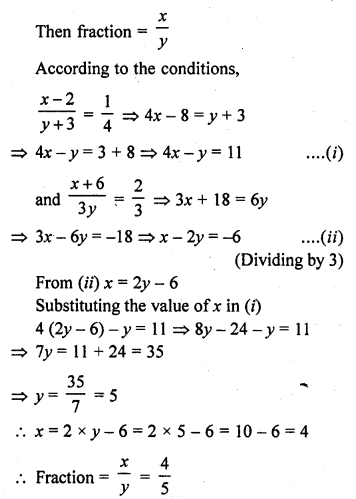Question 7.
The sum of a numerator and denominator of a fraction is 18. If the denominator is increased by 2, the fraction reduces to $$\frac { 1 }{ 3 }$$. Find the fraction. (C.B.S.E. 1997C)
Solution:
Let the numerator of a fractrion = x
and denominator = y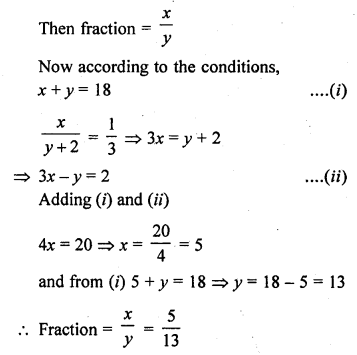Question 8.
If 2 is added to the numerator of a fraction, it reduces to $$\frac { 1 }{ 2 }$$ and if 1 is subtracted from the denominator, it 1 reduces to $$\frac { 1 }{ 3 }$$. Find the fraction. (C.B.S.E. 1997C)
Solution:
Let the numerator of a fraction = x
and denominator = y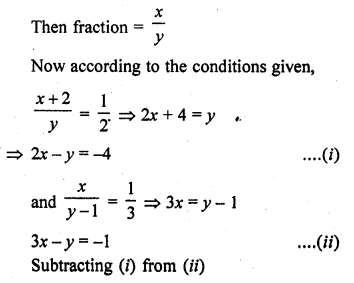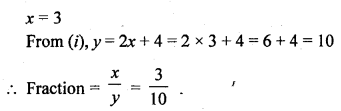Question 9.
The sum of the numerator and denominator of a fraction is 4 more than twice the numerator. If the numerator and denominator are increased by 3, they are in the ratio 2 : 3. Determine the fraction (C.B.S.E. 2001C)
Solution:
Let the numerator of a fraction = x
and denominator = y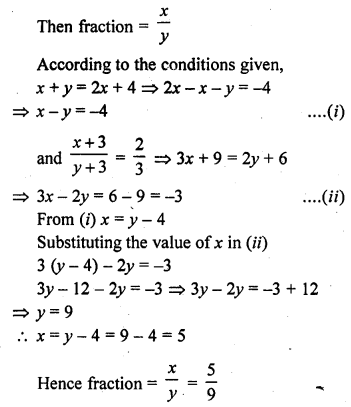Question 10.
If the numerator of a fraction is multiplied by 2 and the denominator is reduced by 5 the fraction becomes $$\frac { 6 }{ 5 }$$. And, if the denominator is doubled and the numerator is increased by 8, the fraction becomes $$\frac { 2 }{ 5 }$$. Find the fraction.
Solution:
Let the numerator of fraction = x
and denominator = y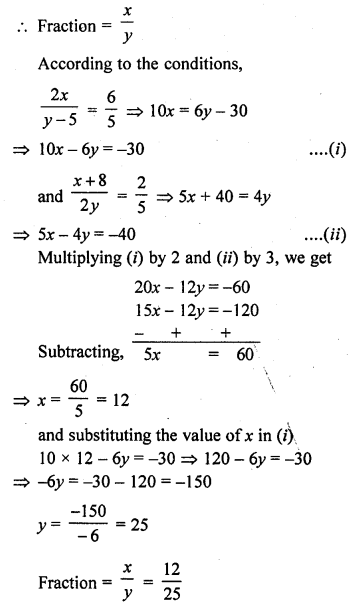Question 11.
The sum of the numerator and denominator of a fraction is 3 less than twice the denominator. If the numerator and denominator are decreased by 1, the numerator becomes half the denominator. Determine the fraction. (C.B.S.E. 2001C)
Solution:
Let the numerator of a fraction = x
and denominator = y
Then fraction = $$\frac { x }{ y }$$
According to the conditions given,
x + y = 2y – 3
=> x + y – 2y = -3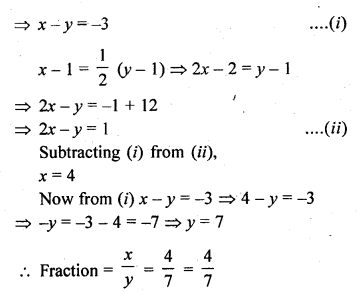Hope given RD Sharma Class 10 Solutions Chapter 3 Pair of Linear Equations in Two Variables Ex 3.8 are helpful to complete your math homework.

If you have any doubts, please comment below. Learn Insta try to provide online math tutoring for you.